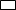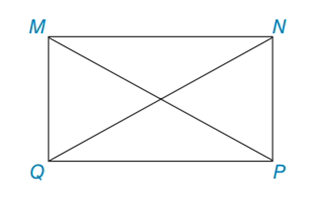Chapter 4.3, Problem 12E### Elementary Geometry for College St...

6th Edition
Daniel C. Alexander + 1 other
ISBN: 9781285195698

#### Solutions

Chapter
Section### Elementary Geometry for College St...

6th Edition
Daniel C. Alexander + 1 other
ISBN: 9781285195698
Textbook Problem
27 views

#In Exercises 11 to 14, consider MNPQ with diagonals M P ¯  and  N Q ¯ . When the answer is not a whole number, leave a square root answer.Exercises 11 – 14If QP = 9 and NP = 6, find NQ and MP.

To determine

To find:

NQ and MP of the given rectangle.

Explanation

The basic property of rectangle:

1) All the properties of a parallelogram apply (the ones that matter here are parallel sides, opposite sides are congruent, and diagonals bisect each other).

2) All angles are right angles by definition.

3) The diagonals are congruent.

Calculation:

Given: QP = 9 and NP = 6

Consider the rectangle.

To find NQ and MP:

From the property, opposite sides are congruent in rectangle.

So, MN = QP = 9

MQ = NP = 6

Let us take right MPQ.

By using Pythagorean Theorem

MP2=QP2+QM2=92+62=81+36=117MP=117

Let us take right QNP

### Still sussing out bartleby?

Check out a sample textbook solution.

See a sample solution

#### The Solution to Your Study Problems

Bartleby provides explanations to thousands of textbook problems written by our experts, many with advanced degrees!

Get Started

#### Simplify the expressions in Exercises 97106. x1/2y2x1/2y

Finite Mathematics and Applied Calculus (MindTap Course List)

#### Write the following numbers in word form. 3. .081

Contemporary Mathematics for Business & Consumers

#### Evaluate 6 8 24 30

Study Guide for Stewart's Multivariable Calculus, 8th

#### By definition the improper integral It is not an improper integral.

Study Guide for Stewart's Single Variable Calculus: Early Transcendentals, 8th